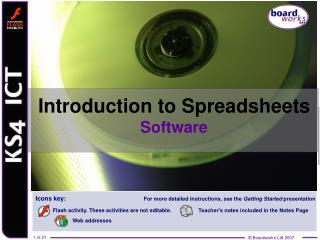Download PresentationIntroduction to Spreadsheets SoftwareIntroduction to Spreadsheets Software - PowerPoint PPT Presentation

Download PresentationIntroduction to Spreadsheets Software
An Image/Link below is provided (as is) to download presentation

Download Policy: Content on the Website is provided to you AS IS for your information and personal use and may not be sold / licensed / shared on other websites without getting consent from its author. While downloading, if for some reason you are not able to download a presentation, the publisher may have deleted the file from their server.

- - - - - - - - - - - - - - - - - - - - - - - - - - - E N D - - - - - - - - - - - - - - - - - - - - - - - - - - -
Presentation Transcript

1. Introduction to SpreadsheetsSoftware

2. Learning objectives • Understand the advantages of using spreadsheets. • Understand the basic formatting functions that can be used with spreadsheets. • Know how to use basic functions and the advantages of displaying data in charts. 2 of 21 © Boardworks Ltd 2007

3. Why use spreadsheets?

4. What does the software do? Spreadsheets can: • do all sorts of calculations, both simple and complex, using formulae • recalculate things quickly when the numbers change • usefunctions to help you set up complex formulae • act as simple databases so that you can sort or search your data • plot charts and graphs • change the appearance of fonts, cell backgrounds and borders • control how many decimal places are shown.

5. Basic features

6. Tracking marks – on paper

7. Tracking marks – on computer The teacher makes a table of names and assignments. What formatting changes could you make to this table so that the information is better presented? Labels Bold font Merged cells Shaded backgrounds Centred text Cell Borders Table border

8. Tracking marks The teacher enters the marks, leaving a blank cell if the student was absent, but putting a 0 if the student did not hand in their work.

9. Using and replicating formulae The teacher can use a formula to work out the average mark for each student… and then replicateit for the other students.

10. Using a function But there’s a problem – can you see what it is? Bethany was absent for one assignment, so her results need to be divided by 4 not 5. The Average function does this automatically.

11. What else can it do?

12. What else can it do? Functions can help the user to: • look up data from lists • do specialized calculations in subjects like finance, statistics and trigonometry • experiment with questions, such as “What if the interest rate went up by 1%?” • uselogic such as IF, AND and OR • change the format of a cell depending on what is in it (conditional formatting) • use pivot tables to summarize data • reduce the number of input errors by setting rules (validation).

13. Nail that function

14. Revise those functions

15. Limitations of tables If we look at the data in a table, it’s quite hard to understand exactly what is happening. The average tells us who is generally getting the best marks, but not who is getting better or worse.

16. Using charts Chartsgive us a picture of our data, and that can help us to see patterns. This chart shows us that Michael is quite consistent (he usually gets about the same mark), and Danny was improving until his last test.

17. Choosing the right chart It is very important to choose the right type of chart if we want to see the patterns in the data clearly. The second chart is plotted from the same data, but doesn’t tell us very much about what is happening.

18. Label the chart

19. Choosing the right chart We don’t always have to show all the information. If we choose only what we want to show, the patterns we see should become clearer. This… is clearer than this

20. Who would use it? Spreadsheets are used by: • engineers to testwhether structures are strong enough • business managers to model their finances • scientists to simulate what might happen in different circumstances • teachers to calculate marks and grades.

21. Summary • Spreadsheet software is mostly used for simple and complex calculations using formulae. • Spreadsheets are also very useful for plotting charts. • They can be used to sort and search data. • Functions make it easier to set up complicated calculations. • Spreadsheet simulations can model situations so that you can ask “What if” questions.